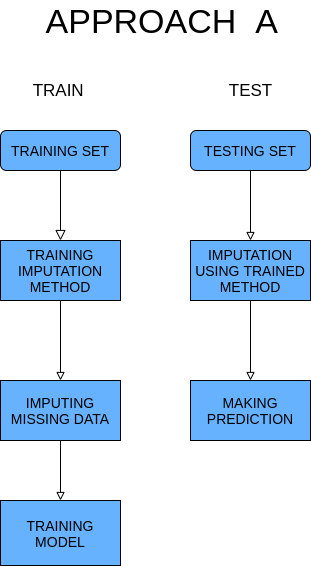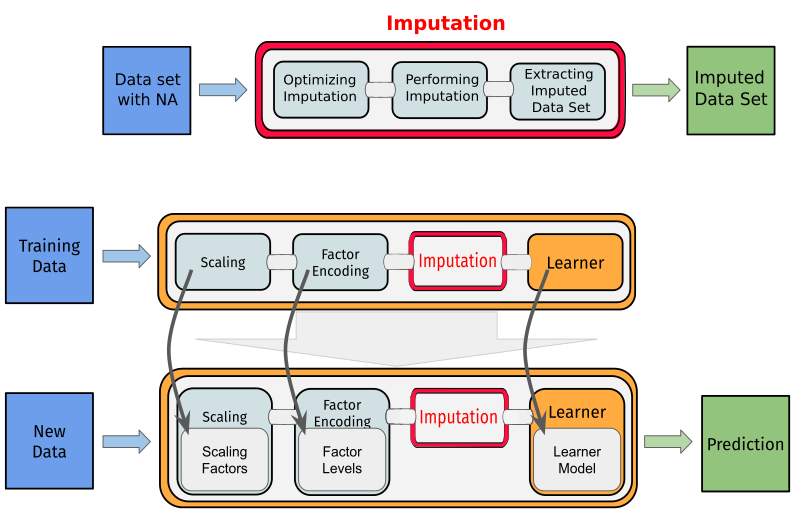In R we have access to a variety of complex methods to impute missing data. For example, we can use complex statistical algorithms like EMB (Expectation–maximization with bootstrap) implemented by Amelia package or machine learning approach in the form of RandomForest implemented by missForest.

Problems appear when u want to use one of these methods in machine learning workflow or just include them in bigger scripts. All of these packages have different implementation for example most of them return different objects. In NADIA we try to automatize the process of using these packages (including available methods to improving imputation). We create uniform interface for the following packages Amelia, mice, missMDA, missForest, missRanger, VIM, softImpute. To allow the user easy access to all methods in machine learning workflow we implemented them as operators in mlr3pipelines (Binder et al. 2020).

## Installation

From Github:

devtools::install_github("https://github.com/ModelOriented/EMMA/", subdir = "NADIA_package/NADIA")

From CRAN:

install.packages("NADIA")

Amelia (Honaker, King, and Blackwell 2011) is a commonly used implementation of Expectation-maximization with bootstrap. By default, this package implements multiple imputations. In the case of mlr3pipelines operators, we have to choose one from produced data sets. Amelia can impute categorical and continuous variables.

mice (van Buuren and Groothuis-Oudshoorn 2011) (Multiple imputation using chain equations) is another popular package to work with missing data. In our implementation, we use linear models to evaluate and improve imputation, mice can be used in two possible approaches.

missMDA (Josse and Husson 2016) package implements methods, especially useful when you want to use PCA or similar after imputation. Because of the number of methods, imputation from this package was separated into two functions:

• The first function implements three complementary methods:

• PCA (Principal Components Analysis) used when data contains only continuous features
• MCA (Multiple Correspondence Analysis) used when data contains only categorical features
• FMAD (Factorial Analysis for Mixed Data) used when data contains mixed features
• Second function implements MFA (Multiple Factor Analysis) which can be used for all types of data.

missForest (Stekhoven and Buehlmann 2012) uses machine learning to impute missing data. In this package, the random-forest model is trained on data with missing values and used to perform imputation.

missRanger (Mayer 2019) is an improved version of missForest where Predictive Mean Matching is added between random-forest iterations. This firstly avoids imputation with values not already present in the original data. Secondly, predictive mean matching tries to raise the variance in the resulting conditional distributions to a realistic level.

VIM (Kowarik and Templ 2016) implements four different imputation methods implemented in separate functions:

• Hot-Deck Data set is sorted and missing values are imputed sequentially running through the data set line (observation) by line (observation). Fast and simple imputation method,
• IRMI (Iterative robust model-based imputation) In each step of the iteration (inner loop), one variable is used as a response variable and the remaining variables serve as the regressors. The procedure is repeated until the algorithm converges (outer loop),
• kNN (k nearest neighbors) An aggregation of the k values of the nearest neighbors is used as an imputed value. Functions used to aggregate neighbors can be pass as arguments,
• Regression Imputer train linear models using column without missing as features and column with missing as a target.

softImpute (Hastie and Mazumder 2015) imputation base on the operation on matrixs. Fast but limited to numeric variables so has to be used alongside some simple imputation method for categorical variables. This imputation function can be pass as an argument.

## Approaches A and B

In standard machine learning, the model is first trained on training data. Then a trained model is used to predict new data. This workflow is recommended and should be used when it’s possible. We call this approach A and present it in the diagram below:Problems start appearing when we want to include advanced imputation methods in this approach. The majority of used packages don’t allow to separated training stage from the imputation stage (expect mice more about this in the next section). Because of this, we have to use something we called approach B. In this case, imputation work separately on training and test sets but the rest of the model is trained the same as in approach A. B approach is presented in the diagram below:Approach B has obvious limitations for example it’s impossible to predict only one example because imputation techniques don’t work for too small samples. Approach B can be beneficial in case when training data has different distributions then testing data. This situation can happen when training is perform using historic data.

## Mice in A approach

Not all included packages are limited to approach B. We can use mice in the A approach using simple tricks. First perform imputation on training data and then use trained imputer on the testing set. To avoid data leak we remove the real values from the testing data set when imputation is performed. These data are added back after imputation. By doing that we allow testing on only one example and avoid all problems with a small test sample size. This approach to mice is available with all mice methods.

## Usage example and mlr3 integration

All included packages are available in form of mlr3pipelines operator so can be used like this:

# Task with missing data from mlr3

# Creating an operator implementing the imputation method
imputation_methods <- PipeOpMice$new() # Imputation task_with_no_missing <- imputation_methods$train(list(task_with_missing))[]

#Check

task_with_missing$missings() #> diabetes age glucose insulin mass pedigree pregnant pressure #> 0 0 5 374 11 0 0 35 #> triceps #> 227  task_with_no_missing$missings()
#> diabetes      age pedigree pregnant  glucose  insulin     mass pressure
#>        0        0        0        0        0        0        0        0
#>  triceps
#>        0

But the real advantage of using NADIA comes from integration with mlr3 (Lang et al. 2019). Because of that, we can easily include advanced imputation techniques inside the machine learning models. For example:

library(mlr3learners)

# Creating graph learner

# imputation method
imp <- PipeOpmissRanger$new() # learner learner <- lrn('classif.glmnet') graph <- imp %>>% learner graph_learner <- GraphLearner$new(graph, id = 'missRanger.learner')
graph_learner$id <- 'missRanger.learner' # resampling set.seed(1) resample(tsk('pima'),graph_learner,rsmp('cv',folds=5)) #> INFO [19:25:49.506] Applying learner 'missRanger.learner' on task 'pima' (iter 4/5) #> INFO [19:25:51.411] Applying learner 'missRanger.learner' on task 'pima' (iter 2/5) #> INFO [19:25:53.553] Applying learner 'missRanger.learner' on task 'pima' (iter 1/5) #> INFO [19:25:54.768] Applying learner 'missRanger.learner' on task 'pima' (iter 3/5) #> INFO [19:25:56.220] Applying learner 'missRanger.learner' on task 'pima' (iter 5/5) #> <ResampleResult> of 5 iterations #> * Task: pima #> * Learner: missRanger.learner #> * Warnings: 0 in 0 iterations #> * Errors: 0 in 0 iterations Advanced imputation technics often can cause errors. NADIA use mlr3’s methods to handle that: # Error handling graph_learner$encapsulate <- c(train='evaluate',predict='evaluate')

data <- iris

data[,1] <- NA

task_problematic <- TaskClassif$new('task',data,'Species') # Resampling # All folds will be tested and the script run further set.seed(1) resample(task_problematic,graph_learner,rsmp('cv',folds=5)) #> INFO [19:26:00.519] Applying learner 'missRanger.learner' on task 'task' (iter 5/5) #> INFO [19:26:00.578] Applying learner 'missRanger.learner' on task 'task' (iter 3/5) #> INFO [19:26:00.646] Applying learner 'missRanger.learner' on task 'task' (iter 4/5) #> INFO [19:26:00.714] Applying learner 'missRanger.learner' on task 'task' (iter 1/5) #> INFO [19:26:00.784] Applying learner 'missRanger.learner' on task 'task' (iter 2/5) #> <ResampleResult> of 5 iterations #> * Task: task #> * Learner: missRanger.learner #> * Warnings: 0 in 0 iterations #> * Errors: 5 in 5 iterations We want to include any form of imputation tuning provided by used packages in our functions. It not possible for every package but it can be used in for example missRanger:  # Turning off encapsulation graph_learner$encapsulate <- c(train='none',predict='none')

# Turning on optimalization
graph_learner$param_set$values$impute_missRanger_B.optimize <- TRUE # Resampling set.seed(1) resample(tsk('pima'),graph_learner,rsmp('cv',folds=5)) #> INFO [19:26:02.186] Applying learner 'missRanger.learner' on task 'pima' (iter 4/5) #> INFO [19:26:06.713] Applying learner 'missRanger.learner' on task 'pima' (iter 2/5) #> INFO [19:26:10.766] Applying learner 'missRanger.learner' on task 'pima' (iter 1/5) #> INFO [19:26:15.222] Applying learner 'missRanger.learner' on task 'pima' (iter 3/5) #> INFO [19:26:19.782] Applying learner 'missRanger.learner' on task 'pima' (iter 5/5) #> <ResampleResult> of 5 iterations #> * Task: pima #> * Learner: missRanger.learner #> * Warnings: 0 in 0 iterations #> * Errors: 0 in 0 iterations Using optimization slows the whole especially in approach B when imputation has to optimize separately on training and test sets. NADIA also implements simple imputation methods like median or mean in approach B. For example:  # Creating graph learner # imputation method imp <- PipeOpMean_B$new()

# learner
learner <- lrn('classif.glmnet')

graph <- imp %>>% learner

graph_learner <- GraphLearner$new(graph) graph_learner$id <-  'mean.learner'
# resampling
set.seed(1)
resample(tsk('pima'),graph_learner,rsmp('cv',folds=5))
#> INFO  [19:26:24.830] Applying learner 'mean.learner' on task 'pima' (iter 4/5)
#> INFO  [19:26:24.992] Applying learner 'mean.learner' on task 'pima' (iter 2/5)
#> INFO  [19:26:25.146] Applying learner 'mean.learner' on task 'pima' (iter 1/5)
#> INFO  [19:26:25.313] Applying learner 'mean.learner' on task 'pima' (iter 3/5)
#> INFO  [19:26:25.466] Applying learner 'mean.learner' on task 'pima' (iter 5/5)
#> <ResampleResult> of 5 iterations
#> * Learner: mean.learner
#> * Warnings: 0 in 0 iterations
#> * Errors: 0 in 0 iterations

NADIA gives the user very easy access to advance imputation techniques scattered on many packages. Also, simplify using these techniques and provide a high level of automatization in using them. Beyond that NADIA implements functions to simulate missing data. This can be especially useful to compare imputation methods with each other.

For example, I will perform two folds cross-validation using missMDA and calculate mean accuracy on simple data set with and without NADIA.

library(missMDA)
library(mlr3learners)

# I can't perform imputation on task so I have to extract data frame

data <- as.data.frame(task$data()) # Splitting into two sets and removing the target column indx <- sample(1:nrow(data),nrow(data)/2) data1 <- data[indx,-1] data2 <- data[-indx,-1] ## Performing imputation with optimization # Features are only numeric so I will use PCA this has to be checked # Optimization ncp1 <- estim_ncpPCA(data1)$ncp

ncp2 <- estim_ncpPCA(data2)$ncp # Imputation data1 <- as.data.frame(imputePCA(data1,ncp1)$completeObs)

data2 <- as.data.frame(imputePCA(data2,ncp2)$completeObs) # Adding back target column data1$diabetes <- data$diabetes[indx] data2$diabetes <- data$diabetes[-indx] # Creating new tasks to make a prediction task1 <- TaskClassif$new("t1",data1,"diabetes")

task2 <- TaskClassif$new("t2",data2,"diabetes") # Training, prediction, and evaluation # Fold1 learner <- lrn("classif.glmnet") learner$train(task1)

p2 <- learner$predict(task2) acc2 <- p2$score(msr("classif.acc"))

# Fold2
learner <- lrn("classif.glmnet")

learner$train(task2) p1 <- learner$predict(task1)

acc1 <- p1$score(msr("classif.acc")) # Mean acc (acc1+acc2)/2 #> classif.acc #> 0.7708333 With NADIA: library(mlr3learners) # Using task form mlr3 task <- tsk("pima") # Imputation, training, prediction, and evaluation graph <- PipeOpMissMDA_PCA_MCA_FMAD$new() %>>% lrns("classif.glmnet")

graph_learner <- GraphLearner$new(graph) graph_learner$id <- 'learner'

#>   0.7682292